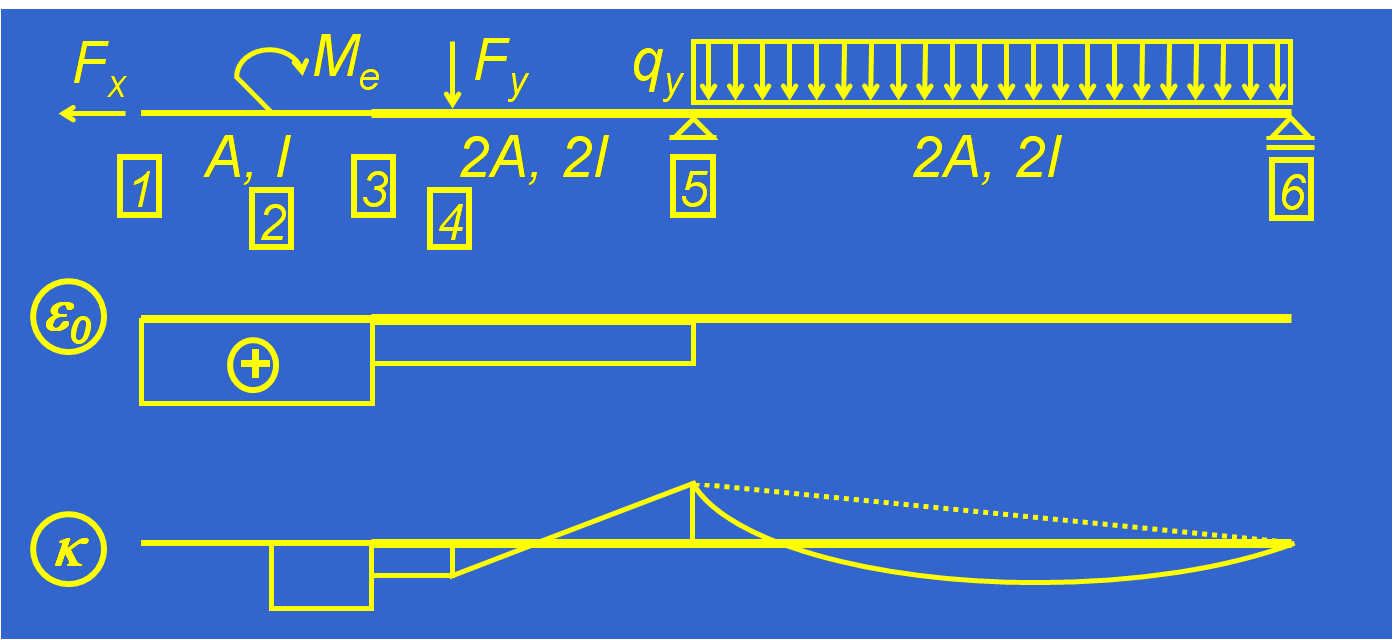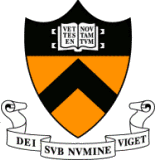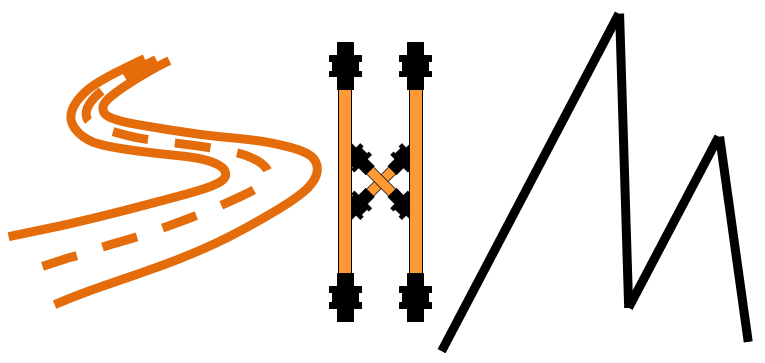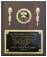BRANKO GLISICHOME
BACKCourse CEE 312: Statics of Structures INTRODUCTION AND OBJECTIVES This course presents the fundamental principles of structural analysis, determination of reactions, internal forces and deflections under the static load conditions. The course is mainly focused to plane structures with line members (beams and trusses) under linear theory hypotheses. Various types of structures are analyzed such as simple beam, continuous beam, frames, arches, suspension bridges, and trusses, including both statically determinate (isostatic) and indeterminate (hyperstatic) structures. Basic principles for construction of influence lines and determination of extreme influences are also presented. Eighty-minute lectures are held twice a week, and eighty-minute precepts are also held twice a week. The lectures and classes are illustrated using examples from practice including the Streicker Bridge at Princeton campus. The prerequisite for the course is CEE 205 Mechanics of Solids. Important: The course is required for concentrators in Structural Engineering and Architecture and Engineering with Structures Focus. Student taking the course will: • Understand “force flow” from load application points through the structure to supports • Learn simple but effective techniques for structural analysis based on graphic statics, decomposition method and energy theorems • Be prepared for the higher level courses in particular for CEE 361 Structural Analysis & Introduction to Finite Element Method and CEE 366 Design of Reinforced Concrete Structures. CURRICULUM 1) Basic notions. Introduction to statics of structures; main hypotheses of linear theory; types of loads; structures, structural members and connections; equilibrium of forces; P1. Graphic statics basics. Two forces with parallel lines of action; two concurrent forces; system of concurrent forces; theorem of three forces; funicular polygon; examples (precepts). 2) Static classification of structures. Degrees of freedom; determinacy and stability of beams; determinacy and stability of structures; examples. 3) Internal loads. Stress distribution in cross-section; Equilibrium of beam segments; Internal forces in a point; S and M functions; N, S, M diagrams; examples. 4) Gerber beam. Simply supported beam with cantilevers; beam with one vertical support; beam with no external supports; examples; a famous “Gereber-like” structure; wrong combinations of elements; challenge. 5) Three hinged structures. Graphic statics; equations of equilibrium; example; beam analogy for vertical loads; two beams with “imaginary” pin supports; two beams with an “imaginary” hinge; improper constraints; complex structures; example; a famous three-hinged structure. 6) Arches and suspension bridges. Indirect load. Three hinged arch; arch with tie; deck stiffened arch; suspension bridge; example; a famous deck-stiffened structure; Masonry arch – challenge. 7) Influence lines, simple structures. Note on sign conventions; influence lines – basic notions; simply supported beam; cantilevered beam; determination of influences using influence lines. 8) Influence lines, complex structures. Gerber beam; arches and suspension bridges; determination of maximum influence using influence lines; examples; a non-famous Gerber beam. 9) Simple trusses: Introduction; determinacy and stability of simple trusses; method of joints; example; zero force members; challenge. 10) Compound and complex trusses: Method of sections; compound trusses; complex trusses; systems of trusses; example; determinacy and stability; example. 11) Influence lines for trusses: Simple truss; systems of trusses; example; challenge. 12) Deflections I: Introduction; sources of deflection, strain, curvature; deflection diagram (elastic curve); main hypothesis and border conditions; qualitative deflection diagram; elastic beam theory and integration method; example. 13) Deflections II: Conjugate-beam method; example; principle of virtual works; example. 14) Indeterminate (hyperstatic) structures – force method: Introduction; advantages and disadvantages of indeterminate structures; methods of analysis; force method; Maxwell’s theorem of reciprocal displacements; example. 15) Symmetric structures and deflections: Symmetric structures; deflections of symmetric structures; example. 16) Influence lines for deformations and indeterminate structures: Influence lines for deformations; example; influence lines for indeterminate structures using force method; example; influence lines using kinematic method; example; deflections; example. SAMPLE READING LIST Structural Analysis (7th Edition), Russell C. Hibbeler, Prentice Hall, 2008 Form and Forces: Designing Efficient, Expressive Structures, Edward Allen, Waclaw Zalewski, John Wiley & Sons, Inc., 2009 Theory of Structures, S. Timoshenko and D.H. Young, McGraw-Hill Book Company, Inc., 1945 COURSE SCHEDULE Lectures: Tuesdays and Thursdays, 08:30AM - 09:50AM, Room E225 Precepts: Mondays and Fridays, 8:30AM - 9:50AM, Room E225 AWARDSCEE 312 was awarded by E-Council for 2010 edition Read more